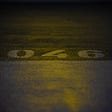# Age Detection using CNN with Keras — with source code

So guys, in today’s blog we will be implementing Age Detection using CNN with the help of Keras. This is going to be a very fun project, So without any further due.

# Code for training the model…

## Step 1 — Import all required libraries.

`import cv2import pandas as pdimport osimport seaborn as snsimport matplotlib.pyplot as pltfrom tensorflow.keras.preprocessing.image import ImageDataGeneratorfrom tensorflow.keras.models import Sequentialfrom tensorflow.keras.layers import Conv2D, Dense, Dropout, Flatten, AveragePooling2D, GlobalAveragePooling2Dfrom tensorflow.keras.callbacks import ModelCheckpointimport numpy as npfrom sklearn.model_selection import train_test_splitfrom keras.utils import np_utilsnp.random.seed(42)tf.random.set_seed(42)`

## Step 2 — Read the input images and extract their labels.

`all_images = os.listdir('combined_faces/')ranges = ['1-2','3-9','10-20','21-27','28-45','46-65','66-116']X = []y = []l = len(all_images)for a in range(l):    X.append(cv2.imread(f'combined_faces/{all_images[a]}',0))    age = int(all_images[a].split('_'))        if age>=1 and age<=2:        y.append(0)    elif age>=3 and age<=9:        y.append(1)    elif age>=10 and age<=20:        y.append(2)    elif age>=21 and age<=27:        y.append(3)    elif age>=28 and age<=45:        y.append(4)    elif age>=46 and age<=65:        y.append(5)    elif age>=66 and age<=116:        y.append(6)    print(str(a)+'/'+str(l))np.savez_compressed('compressed image data.npz',x=X,y=y)`
• Here we are reading our images in grayscale mode and storing them in X array.
• We are storing their ages in the y array.
• And finally, we are saving X and y arrays in npz compressed format so that we don’t need to read images again and again.

How to load the compressed npz data…

`loaded = np.load('compressed image data.npz')X = loaded['x']y = loaded['y']`

## Step 3 — Visualize an image.

`plt.imshow(X,cmap=’gray’)`

## Step 4 — One hot encode y array.

`y = np_utils.to_categorical(y)y`

## Step 5 — Train Test Split the data for creating Age Detection using the CNN model.

`X_train, X_test, y_train, y_test = train_test_split(X, y, test_size=0.30, random_state=42)X_train = np.array(X_train).reshape(-1,200,200,1)X_test = np.array(X_test).reshape(-1,200,200,1)`
• We are train test splitting the data here and reshaping X in the correct format to feed it in the model in further steps.

## Step 6 — Initialize some constants.

`IMG_HEIGHT = 200IMG_WIDTH = 200IMG_SIZE = (IMG_HEIGHT,IMG_WIDTH)batch_size = 128epochs = 60`

## Step 7 — Create ImageDataGenerator objects for data augmentation.

`train_datagen = ImageDataGenerator(rescale=1./255,                                   horizontal_flip=True,                                   shear_range=0.2,                                   zoom_range=0.2,                                   height_shift_range=0.1,                                   width_shift_range=0.1,                                   rotation_range=15)test_datagen = ImageDataGenerator(rescale=1./255)`

## Step 8 — Augment the Data for Age Detection using the CNN model.

`train_data = train_datagen.flow(X_train,y_train,batch_size)test_data = test_datagen.flow(X_test,y_test,batch_size)`
• Finally augmenting the data using flow.

## Step 9 — Creating the Age Detection using the CNN model.

`final_cnn = Sequential()final_cnn.add(Conv2D(filters=32, kernel_size=3, activation='relu', input_shape=(200, 200, 1)))    # 3rd dim = 1 for grayscale images.final_cnn.add(AveragePooling2D(pool_size=(2,2)))final_cnn.add(Conv2D(filters=64, kernel_size=3, activation='relu'))final_cnn.add(AveragePooling2D(pool_size=(2,2)))final_cnn.add(Conv2D(filters=128, kernel_size=3, activation='relu'))final_cnn.add(AveragePooling2D(pool_size=(2,2)))final_cnn.add(Conv2D(filters=256, kernel_size=3, activation='relu'))final_cnn.add(AveragePooling2D(pool_size=(2,2)))final_cnn.add(GlobalAveragePooling2D())final_cnn.add(Dense(132, activation='relu'))final_cnn.add(Dense(7, activation='softmax'))final_cnn.compile(loss='categorical_crossentropy', optimizer='adam', metrics=['accuracy'])final_cnn.summary()`
• Here we have created and compiled our model.

## Step 10 — Create a Model Checkpoint to save just the best model.

`checkpoint = ModelCheckpoint(filepath="final_cnn_model_checkpoint.h5",                             monitor='val_accuracy',                             save_best_only=True,                             save_weights_only=False,                             verbose=1                            )`
• ModelCheckpoint is just a simple callback in Keras which says that store only the best model.
• The best model means the model with the highest val_acuracy here.

## Step 11 — Train the Age Detection using the CNN model.

`history = final_cnn.fit(train_data,                    batch_size=batch_size,                    validation_data=test_data,                    epochs=epochs,                    callbacks=[checkpoint],                    shuffle=False                        )`

## Step 12 — Visualize the training process.

`plotting_data_dict = history.historyplt.figure(figsize=(12,8))test_loss = plotting_data_dict['val_loss']training_loss = plotting_data_dict['loss']test_accuracy = plotting_data_dict['val_accuracy']training_accuracy = plotting_data_dict['accuracy']epochs = range(1,len(test_loss)+1)plt.subplot(121)plt.plot(epochs,test_loss,marker='X',label='test_loss')plt.plot(epochs,training_loss,marker='X',label='training_loss')plt.legend()plt.subplot(122)plt.plot(epochs,test_accuracy,marker='X',label='test_accuracy')plt.plot(epochs,training_accuracy,marker='X',label='training_accuracy')plt.legend()plt.savefig('training.png')`

# Code for live webcam prediction of Age Detection using CNN…

`import cv2import imutilsimport numpy as npfrom tensorflow.keras.models import load_modelmodel = load_model('age_detect_cnn_model.h5')detector = cv2.CascadeClassifier('haarcascade_frontalface_default.xml')ranges = ['1-2','3-9','10-20','21-27','28-45','46-65','66-116']cam = cv2.VideoCapture(0)while 1:    ret,frame = cam.read()    if ret:        faces = detector.detectMultiScale(frame,1.3,5)        for x,y,w,h in faces:            face = frame[y:y+h,x:x+w]            face = cv2.cvtColor(face,cv2.COLOR_BGR2GRAY)            face = cv2.resize(face,(200,200))            face = face.reshape(1,200,200,1)            age = model.predict(face)            cv2.rectangle(frame,(x,y),(x+w,y+h),(255,0,0),2)            cv2.rectangle(frame,(x,y+h),(x+w,y+h+50),(255,0,0),-1)            cv2.putText(frame,ranges[np.argmax(age)],(x+65,y+h+35),cv2.FONT_HERSHEY_DUPLEX,0.8,(255,255,255),2)                    cv2.imshow('Live',frame)                    if cv2.waitKey(1)==27:        breakcam.release()cv2.destroyAllWindows()`
• Line 1–4 — Importing required libraries.
• Line 6 — Loading the age detector model we trained above.
• Line 8 — Using Haarcascades to detect a face in the frame.
• Line 10 — Creating an age range array on which we trained our model.
• Line 12 — Initiating VideoCapture object to access the webcam.
• Line 14–32 — Reading image from webcam, detecting face, cropping out face, grayscaling the face, resizing the face, reshaping it to (1,200,200,1) format which is required by Keras models to predict, and then finally predicting the age range and showing it on the final image.
• Line 34–35 — Release the camera object and destroy all windows.

# Final Results of Age Detection using CNN…

Do let me know if there’s any query regarding Age Detection using CNN by contacting me on email or LinkedIn.

To explore more Machine Learning, Deep Learning, Computer Vision, NLP, Flask Projects visit my blog — Machine Learning Projects

For further code explanation and source code visit here https://machinelearningprojects.net/age-detection-using-cnn-with-keras/

So this is all for this blog folks, thanks for reading it and I hope you are taking something with you after reading this and till the next time 👋…

Read my previous post: CATS AND DOGS CLASSIFIER — EASIEST WAY

--

--

--

## More from MLearning.ai

Data Scientists must think like an artist when finding a solution when creating a piece of code. ⚪️ Artists enjoy working on interesting problems, even if there is no obvious answer ⚪️ linktr.ee/mlearning 🔵 Follow to join our 18K+ Unique DAILY Readers 🟠

## Dog Breed Classifier — Power of Neural Networks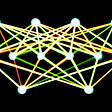## Two Resampling Approaches to Assess a Model: Cross-validation and Bootstrap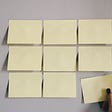## Recommender System: Ranking Algorithms and Training Architectures## NLP: Building a Basic “Automated Text Filler”- An Introduction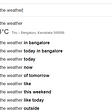## Dialog Flow Analysis Notebook in Cloud Pak For Data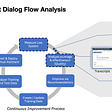## There’s LIGHT even in the DARKEST places## Google’s 7 steps of Machine Learning in practice: a TensorFlow example for structured data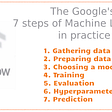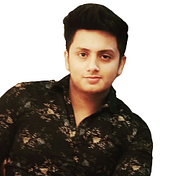## Abhishek Sharma

Data Scientist || Blogger || machinelearningprojects.net

## HOW TO GENERATE A NEGATIVE IMAGE IN PYTHON USING OPENCV — INTERESTING PROJECT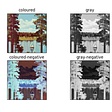## Detecting Melanoma Skin Cancer with Computer Vision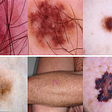## A Simple Introduction to Object Detection## Library for SVHN Dataset